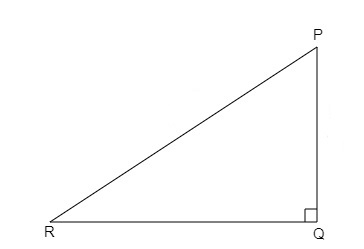# In $\triangle PQR$, right angled at $Q, PQ = 4\ cm$ and $RQ = 3\ cm$. Find the values of $sin\ P, sin\ R, sec\ P$ and $sec\ R$.

Given:

In $\triangle PQR$, right angled at $Q, PQ = 4\ cm$ and $RQ = 3\ cm$.

To do:

We have to find the values of $sin\ P, sin\ R, sec\ P$ and $sec\ R$.

Solution:We know that,

In a right-angled triangle $PQR$ with right angle at $Q$,

By Pythagoras theorem,

$PR^2=PQ^2+QR^2$

By trigonometric ratios definitions,

$sin\ P=\frac{Opposite}{Hypotenuse}=\frac{QR}{PR}$

$sin\ R=\frac{Opposite}{Hypotenuse}=\frac{PQ}{PR}$

$sec\ P=\frac{Hypotenuse}{Adjacent}=\frac{PR}{PQ}$

$sec\ R=\frac{Hypotenuse}{Adjacent}=\frac{PR}{QR}$

Here, $PQ = 4\ cm$ and $RQ = 3\ cm$.

$PR^2=PQ^2+QR^2$

$\Rightarrow PR^2=(4)^2+(3)^2$

$\Rightarrow PR^2=16+9$

$\Rightarrow PR=\sqrt{25}=5$

Therefore,

$sin\ P=\frac{QR}{PR}=\frac{3}{5}$

$sin\ R=\frac{PQ}{PR}=\frac{4}{5}$

$sec\ P=\frac{PR}{PQ}=\frac{5}{4}$

$sec\ R=\frac{PR}{QR}=\frac{5}{3}$

Updated on: 10-Oct-2022

45 Views